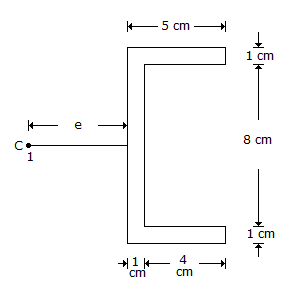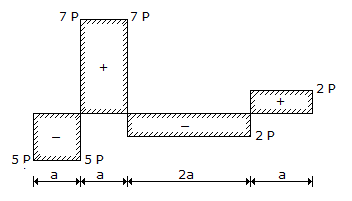# Civil Engineering - UPSC Civil Service Exam Questions

21.

A cold drawn seamless steel tubing subject to internal pressure, has a diameter of 6 cm and wall thickness of 0.2 cm. The ultimate strength of steel is 3600 kg/cm2. The bursting pressure (in kg/cm2) is

 A. 120 B. 240 C. 480 D. 960

Explanation:

No answer description available for this question. Let us discuss.

22.

Which of the following factors are associated with the behaviour of sand mass during earthquake to cause liquefaction ?
1. Number of stress cycles
2. The frequency, and amplitude of vibration of waves generated by an earthquake
3. Characteristics of sand
4. Relative density
Select the correct answer using the codes given below :

 A. 1, 2 and 3 B. 1, 2, 3 and 4 C. 2 and 4 D. 3 and 4

Explanation:

No answer description available for this question. Let us discuss.

23.

The ratio of total elongation of a bar of uniform cross-section produced under its own weight to the elongation produced by an external load equal to the weight of the bar is

 A. 2 B. 1 C. 1/2 D. 1/4

Explanation:

No answer description available for this question. Let us discuss.

24.

The figure refers to a channel section of uniform thickness of 1 cm used as a beam with the cross-sectional dimensions shown in cm units. If the Moment of Inertia of channel Ixx be equal to 246 cm4 and C be the shear centre, then the value of e in cm is equal toA. 1.5 B. 2.5 C. 2.25 D. 1.67

Explanation:

No answer description available for this question. Let us discuss.

25.

The shear force diagram of a beam is shown in the given figure. The absolute maximum bending moment in the beam isA. (2P x a) B. (5P x a) C. (4P x a) D. (7P x a)